# ISEE Upper Level Quantitative : How to find the length of a chord

## Example Questions

### Example Question #1 : How To Find The Length Of A Chord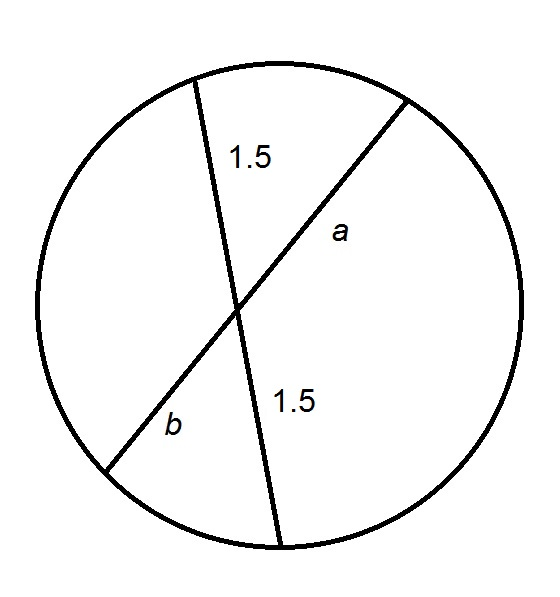Refer to the above figure. Which is the greater quantity?

(a)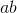(b) 3

(a) and (b) are equal

(b) is the greater quantity

It is impossible to determine which is greater from the information given

(a) is the greater quantity

(b) is the greater quantity

Explanation:

If two chords intersect inside a circle, both chords are cut in a way such that the products of the lengths of the two chords formed in each are the same - in other words,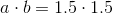or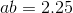Therefore,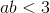.

### Example Question #2 : How To Find The Length Of A Chord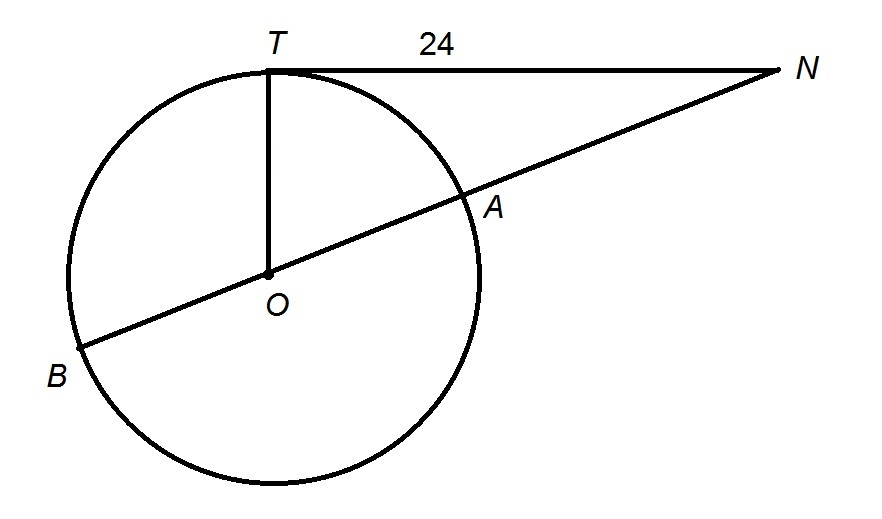Figure NOT drawn to scale

In the above figure,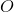is the center of the circle, and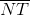is a tangent to the circle. Also, the circumference of the circle is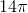.

Which is the greater quantity?

(a)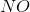(b) 25

It is impossible to determine which is greater from the information given

(a) and (b) are equal

(b) is the greater quantity

(a) is the greater quantity

(a) and (b) are equal

Explanation: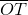is a radius of the circle from the center to the point of tangency of, so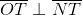,

and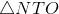is a right triangle. The length of legis known to be 24. The other legis a radius radius; we can find its length by dividing the circumference by: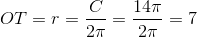The length hypotenuse,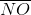, can be found by applying the Pythagorean Theorem: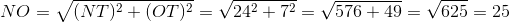.

### Example Question #3 : How To Find The Length Of A Chord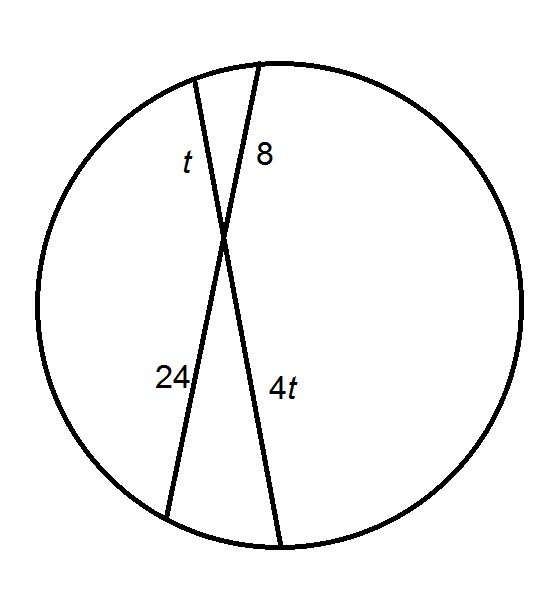Figure NOT drawn to scale.

Refer to the above figure. Which is the greater quantity?

(a)(b) 7

(a) and (b) are equal

(a) is the greater quantity

It is impossible to determine which is greater from the information given

(b) is the greater quantity

(b) is the greater quantity

Explanation:

If two chords intersect inside a circle, both chords are cut in a way such that the products of the lengths of the two chords formed in each are the same - in other words,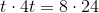Solving for: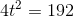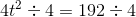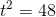Since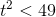, it follows that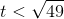, or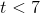.

### Example Question #4 : How To Find The Length Of A Chord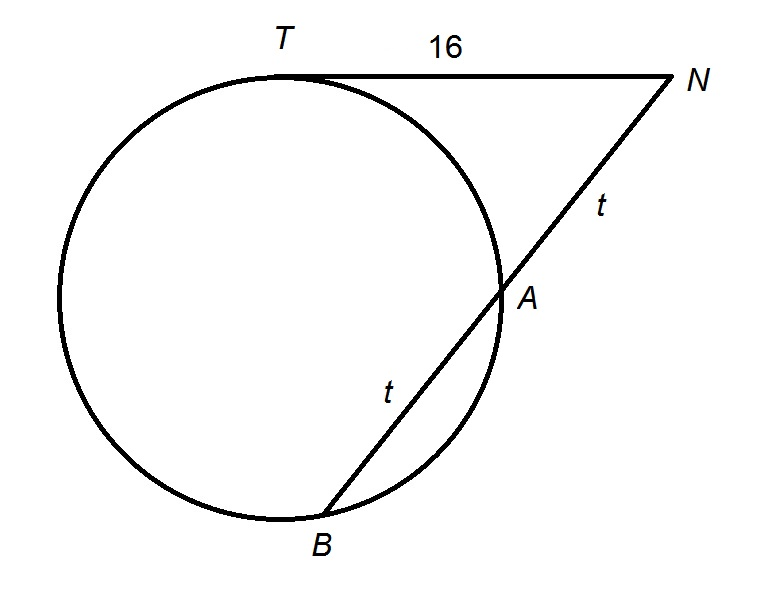In the above figure,is a tangent to the circle.

Which is the greater quantity?

(a)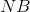(b) 32

(a) is the greater quantity

(a) and (b) are equal

(b) is the greater quantity

It is impossible to determine which is greater from the information given

(b) is the greater quantity

Explanation:

If a secant segment and a tangent segment are constructed to a circle from a point outside it, the square of the distance to the circle along the tangent is equal to the product of the distances to the two points on the circle along the secant; in other words,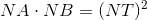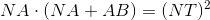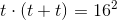Simplifying, then solving for: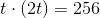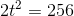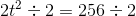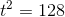To compareto 32, it suffices to compare their squares: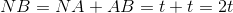, so, applying the Power of a Product Principle, then substituting,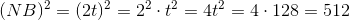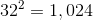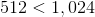, so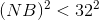it follows that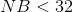.

### Example Question #5 : How To Find The Length Of A Chord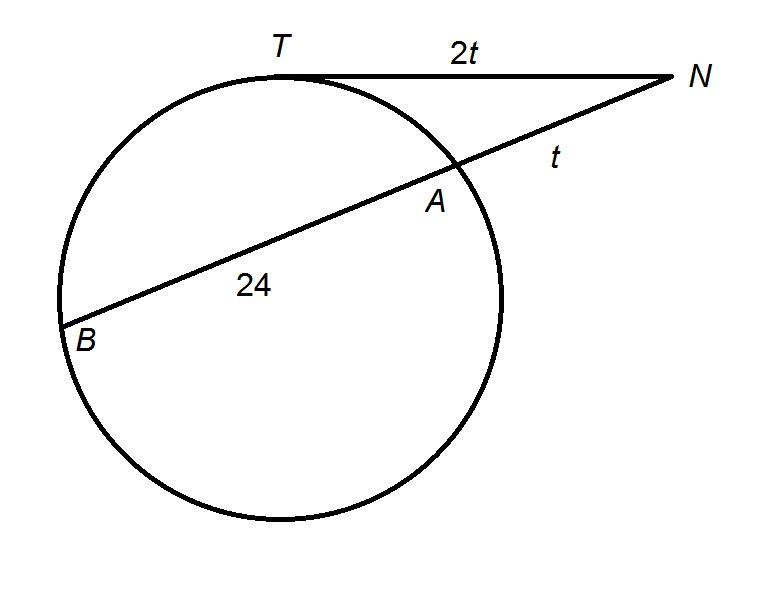Figure NOT drawn to scale

In the above figure,is a tangent to the circle.

Which is the greater quantity?

(a)(b) 8

(b) is the greater quantity

It is impossible to determine which is greater from the information given

(a) and (b) are equal

(a) is the greater quantity

(a) and (b) are equal

Explanation:

If a secant segment and a tangent segment are constructed to a circle from a point outside it, the square of the distance to the circle along the tangent is equal to the product of the distances to the two points on the circle intersected by the secant; in other words,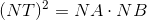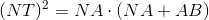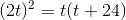Simplifying and solving for: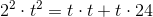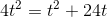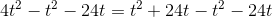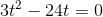Factoring out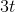: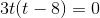Either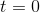- which is impossible, sincemust be positive, or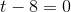, in which case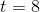.

### Example Question #1 : Circles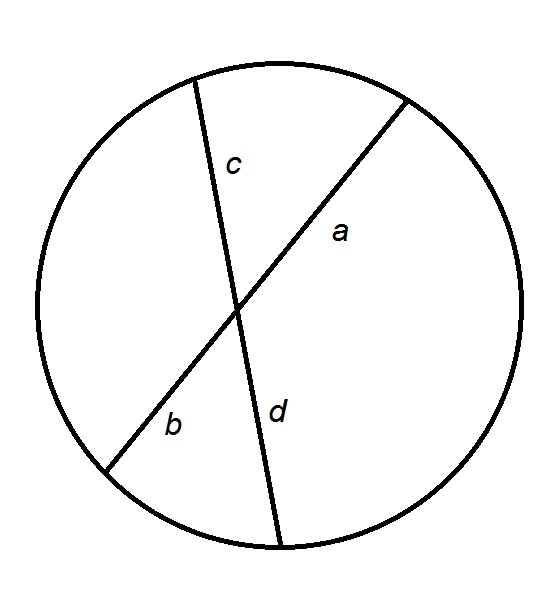Refer to the above figure. Which is the greater quantity?

(a)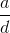(b)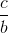(b) is the greater quantity

(a) and (b) are equal

It is impossible to determine which is greater from the information given

(a) is the greater quantity

(a) and (b) are equal

Explanation:

If two chords intersect inside a circle, both chords are cut in a way such that the products of the lengths of the two chords formed in each are the same - in other words,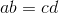Divide both sides of this equation by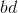, then cancelling: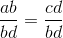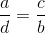The two quantities are equal.